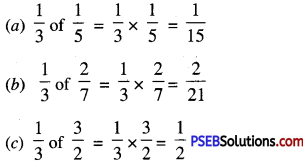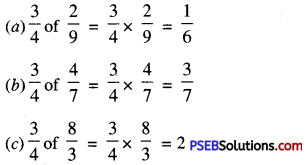# PSEB 7th Class Maths Solutions Chapter 2 Fractions and Decimals Ex 2.3

Punjab State Board PSEB 7th Class Maths Book Solutions Chapter 2 Fractions and Decimals Ex 2.3 Textbook Exercise Questions and Answers.

## PSEB Solutions for Class 7 Maths Chapter 2 Fractions and Decimals Ex 2.3

1. Solve the following :

Question (i).
$$\frac {1}{3}$$ of
(a) $$\frac {1}{5}$$
(b) $$\frac {2}{7}$$
(c) $$\frac {3}{2}$$Question (ii).
$$\frac {3}{4}$$ of
(a) $$\frac {2}{9}$$
(b) $$\frac {4}{7}$$
(c) $$\frac {8}{3}$$2. Multiply the fractions and reduce it to the lowest form (If possible) :

Question (i).
$$\frac{2}{7} \times \frac{7}{9}$$
$$\frac{2}{7} \times \frac{7}{9}$$
= $$\frac {2}{9}$$

Question (ii).
$$\frac{1}{3} \times \frac{15}{8}$$
$$\frac{1}{3} \times \frac{15}{8}$$
= $$\frac {5}{8}$$

Question (iii).
$$\frac{12}{27} \times \frac{3}{9}$$
$$\frac{12}{27} \times \frac{3}{9}$$
= $$\frac {4}{27}$$

Question (iv).
$$\frac{2}{5} \times \frac{6}{4}$$
$$\frac{2}{5} \times \frac{6}{4}$$
= $$\frac {3}{5}$$

Question (v).
$$\frac{81}{100} \times \frac{6}{7}$$
$$\frac{81}{100} \times \frac{6}{7}$$
= $$\frac {243}{350}$$

Question (vi).
$$\frac{3}{5} \times \frac{5}{27}$$
$$\frac{3}{5} \times \frac{5}{27}$$
= $$\frac {1}{9}$$3. Multiply the following fractions :

Question (i).
$$\frac{3}{2} \times 5 \frac{1}{3}$$
$$\frac{3}{2} \times 5 \frac{1}{3}$$
= $$\frac{3}{2} \times \frac{16}{3}$$
= 8

Question (ii).
$$\frac{1}{7} \times 5 \frac{2}{3}$$
$$\frac{1}{7} \times 5 \frac{2}{3}$$
= $$\frac{1}{7} \times \frac{17}{3}$$
= $$\frac {17}{21}$$

Question (iii).
$$2 \frac{5}{6} \times 4$$
$$2 \frac{5}{6} \times 4$$
= $$\frac {17}{6}$$ × 4
= $$\frac {34}{3}$$
= 11$$\frac {1}{3}$$

Question (iv).
$$4 \frac{1}{3} \times 9 \frac{1}{4}$$
$$4 \frac{1}{3} \times 9 \frac{1}{4}$$
= $$\frac{13}{3} \times \frac{37}{4}$$
= $$\frac {481}{12}$$

Question (v).
$$2 \frac{2}{3} \times 3 \frac{5}{8}$$
$$2 \frac{2}{3} \times 3 \frac{5}{8}$$
= $$\frac{8}{3} \times \frac{29}{8}$$
= 9$$\frac {2}{3}$$

Question (vi).
$$3 \frac{1}{5} \times 2 \frac{1}{4}$$
$$3 \frac{1}{5} \times 2 \frac{1}{4}$$
= $$\frac{16}{5} \times \frac{9}{4}$$
= $$\frac {36}{5}$$
= 7$$\frac {1}{5}$$

4. Which fraction is greater in the following fractions ?

Question (i).
$$\frac {3}{2}$$ of $$\frac {2}{7}$$ or $$\frac {5}{2}$$ of $$\frac {3}{8}$$
$$\frac {3}{2}$$ of $$\frac {2}{7}$$
= $$\frac{3}{2} \times \frac{2}{7}$$
= $$\frac {3}{7}$$
or
$$\frac {5}{2}$$ of $$\frac {3}{8}$$
= $$\frac{5}{2} \times \frac{3}{8}$$
= $$\frac {15}{16}$$
Since, $$\frac {15}{16}$$ > $$\frac {3}{7}$$
So, $$\frac {5}{2}$$ of $$\frac {3}{8}$$ is greater.

Question (ii).
$$\frac {1}{2}$$ of $$\frac {6}{5}$$ or $$\frac {1}{3}$$ of $$\frac {4}{5}$$
$$\frac {1}{2}$$ of $$\frac {6}{5}$$
= $$\frac {1}{2}$$ × $$\frac {6}{5}$$
= $$\frac {3}{5}$$
or
$$\frac {1}{3}$$ of $$\frac {4}{5}$$
= $$\frac {1}{3}$$ × $$\frac {4}{5}$$
= $$\frac {4}{15}$$
Since, $$\frac {3}{5}$$ > $$\frac {4}{15}$$
So, $$\frac {1}{2}$$ of $$\frac {6}{5}$$ is greater.5. If the speed of a car is 105 $$\frac {1}{3}$$ km/hr, find the distance covered by it m 3$$\frac {2}{3}$$ hours.
Distance travelled in 1 hour= 105$$\frac {1}{3}$$ km
= $$\frac {316}{3}$$ km
Distance travelled in 3$$\frac {2}{3}$$ hours i.e. in $$\frac {11}{3}$$ hours
= $$\frac {11}{3}$$ × $$\frac {316}{3}$$ km
= $$\frac {3476}{9}$$ km
= 386$$\frac {2}{9}$$km

6. The length of a rctangular plot of land is 29$$\frac {3}{7}$$ m. If its breadth is 12$$\frac {8}{11}$$m, find its area.
Length of rectangular plot (l)
= 29$$\frac {3}{7}$$m = $$\frac {206}{7}$$m
= 12$$\frac {8}{11}$$m = $$\frac {140}{11}$$m
Area of rectangular plot
= l × b = $$\frac{206}{7} \mathrm{~m} \times \frac{140}{11} \mathrm{~m}$$
= $$\frac{4120}{11}$$ sq.m
= 374$$\frac{6}{11}$$ sq.m

7. If a cloth costs ₹ 120 $$\frac {1}{4}$$ per metre, find the cost of 4$$\frac {1}{3}$$ metre of this cloth.
Per m cost of cloth = ₹ 120$$\frac {1}{4}$$
= ₹ $$\frac {481}{4}$$
Cost of 4$$\frac {1}{3}$$ metre of this cloth
= $$\frac {1}{3}$$ × ₹ $$\frac {481}{4}$$
= ₹ $$\frac {6253}{12}$$
= ₹ 521$$\frac {1}{12}$$8. Multiple choice questions :

Question (i).
$$\frac {1}{4}$$ of $$\frac {8}{3}$$ is :
(a) $$\frac {9}{7}$$
(b) $$\frac {8}{4}$$
(c) $$\frac {2}{3}$$
(d) 1
(c) $$\frac {2}{3}$$

Question (ii).
$$\frac {3}{2}$$ × $$\frac {2}{3}$$ = ?
(a) 1
(b) $$\frac {5}{6}$$
(c) 3
(d) $$\frac {6}{5}$$
(a) 1

Question (iii).
The value of the product of two proper fractions is :
(a) greater than both of the proper fractions.
(b) lesser than both of the proper fractions.
(c) lie between the given two fractions.
(d) none of these.
(b) lesser than both of the proper fractions.9. Check if the following equations are ‘True’ or ‘False’ :

Question (i).
$$1 \frac{2}{3} \times 4 \frac{5}{7}=4 \frac{10}{21} ?$$ (True/False)
$$\frac{3}{4} \times \frac{2}{3}=\frac{2}{3} \times \frac{3}{4} ?$$ (True/False)